### Home > CAAC > Chapter 8 > Lesson 8.2.1 > Problem8-49

8-49.
1. For each rule represented below, state the x- and y-intercepts. Homework Help ✎

1.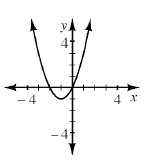2.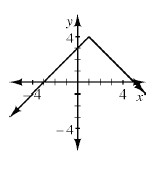3.  x −3 −2 −1 0 1 2 3 y 8 3 0 −1 0 3 8
4. 2x + 3y = 18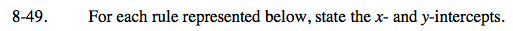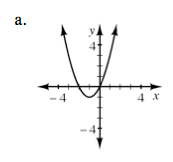Where does the relation intersect the y-axis? the x-axis? Does it intersect with the same axis multiple times?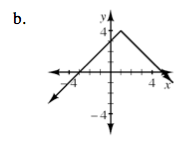y-intercept: (0, 3)
x-intercepts: (−3, 0) (5, 0)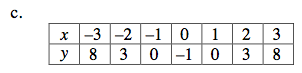Look for the zeroes in the table.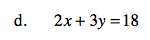To find the y-intercept, set x = 0.
To find the x-intercept, set y = 0.